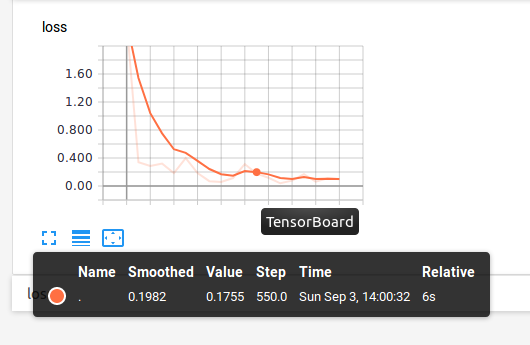# Tensorflow 9: MNIST 2

My previous post. .  All my code can be found on github (9_MNIST_2.ipynb)

In the last guide we had a single hidden layer Neural Network and achieved 86% accuracy.

Now we want to try and increase the accuracy by adding an additional hidden layer.

We will have the exact same network as previously except we will now have 2 hidden layers of 256 nodes each.

#### Network Architecture

Firstly the network parameters are described then the training parameters.  Then i will create weights and biases for the different layers.  Then link them all up.

```#Network Parameters
INPUTS = 784       # 28 x 28 = 784 input pixels
HIDDEN_1 = 256     # we will start with a NN with 1 hidden layer and 40 nodes.
HIDDEN_2 = 256     # Second layer added
OUTPUTS = 10       # 10 possible outputs - 0->9

#Training Parameters
epochs = 10000
display_epochs = 50
batch_size = 100
learning_rate = 0.001

#Structure
# Weights - Input to Hidden 1
weight1 = tf.random_normal([INPUTS, HIDDEN_1])
weight1 = tf.Variable(weight1, name='W1')

bias1 = tf.random_normal([HIDDEN_1])
bias1 = tf.Variable(bias1, name='B1')

#Weights - Hidden 1 to Hidden 2
weight2 = tf.random_normal([HIDDEN_1, HIDDEN_2])
weight2 = tf.Variable(weight2, name='W2')

bias2 = tf.random_normal([HIDDEN_2])
bias2 = tf.Variable(bias2, name='B2')

# Weights - Hidden 2 to Output
weight3 = tf.random_normal([HIDDEN_2, OUTPUTS])
weight3 = tf.Variable(weight3, name='W2')

bias3 = tf.random_normal([OUTPUTS])
bias3 = tf.Variable(bias3, name='B2')

#input to hidden 1

#hidden 1 to hidden 2

#hidden2 to output

#apply final activation
result = tf.nn.softmax(y)

#loss and training
loss = tf.reduce_mean(tf.nn.softmax_cross_entropy_with_logits(logits=y, labels=y_data))
train = optimizer.minimize(loss)```

We train this the same as previously with the exact same code and using the same accuracy code we now get 95%.  That’s an good accuracy increase.#### Simplify Code

The code is starting to balloon out and look complicated for what is quite a simple network.  Imagine how it would look with 8 layers or 15.

Lets use some built in higher level Tensorflow operations to simplify the code.  Rewrite it as follows:

```def multilayer_perceptron2(x):
fc1 = tf.contrib.layers.fully_connected(x, HIDDEN_1, activation_fn=tf.nn.relu)
fc2 = tf.contrib.layers.fully_connected(fc1, HIDDEN_2, activation_fn=tf.nn.relu)
out = tf.contrib.layers.fully_connected(fc2, OUTPUT, activation_fn=None)
return out

y = multilayer_perceptron2(x_data)
```

Result:The tf.contrib.fully_connected creates a fully connected layer where you define the input tensor, output size and activation function and it links everything up for you. This is great if you are not doing anything unusual.  In the image below from Tensorboard you can see the weights, biases, matmul, addition and activation relu are all automatically generated.These can also handle max pooling and convolutional layers we will learn about soon.

Since the accuracy improved by adding more layer could we just keep on adding more nodes and layers?  There are some issues to consider with this approach. Firstly the more layers and nodes you add, the more weights and biases you need to store and train. This means more memory usage and more training time required.  And simply adding more nodes and layers will not guarantee results or improvements.

Lets add more layers and see the effect it has.  Lets go with 5 layers

```def multilayer_perceptron5(x):
fc1 = tf.contrib.layers.fully_connected(x, HIDDEN_1, activation_fn=tf.nn.relu)
fc2 = tf.contrib.layers.fully_connected(fc1, HIDDEN_2, activation_fn=tf.nn.relu)
fc3 = tf.contrib.layers.fully_connected(fc2, HIDDEN_2, activation_fn=tf.nn.relu)
fc4 = tf.contrib.layers.fully_connected(fc3, HIDDEN_2, activation_fn=tf.nn.relu)
fc5 = tf.contrib.layers.fully_connected(fc4, HIDDEN_2, activation_fn=tf.nn.relu)
out = tf.contrib.layers.fully_connected(fc5, OUTPUTS, activation_fn=None)
return out```Lets go with 10 layers with more training (100,000).

```def multilayer_perceptron10(x):
fc1 = tf.contrib.layers.fully_connected(x, HIDDEN_1, activation_fn=tf.nn.relu)
fc2 = tf.contrib.layers.fully_connected(fc1, HIDDEN_2, activation_fn=tf.nn.relu)
fc3 = tf.contrib.layers.fully_connected(fc2, HIDDEN_2, activation_fn=tf.nn.relu)
fc4 = tf.contrib.layers.fully_connected(fc3, HIDDEN_2, activation_fn=tf.nn.relu)
fc5 = tf.contrib.layers.fully_connected(fc4, HIDDEN_2, activation_fn=tf.nn.relu)
fc6 = tf.contrib.layers.fully_connected(fc5, HIDDEN_2, activation_fn=tf.nn.relu)
fc7 = tf.contrib.layers.fully_connected(fc6, HIDDEN_2, activation_fn=tf.nn.relu)
fc8 = tf.contrib.layers.fully_connected(fc7, HIDDEN_2, activation_fn=tf.nn.relu)
fc9 = tf.contrib.layers.fully_connected(fc8, HIDDEN_2, activation_fn=tf.nn.relu)
fc10 = tf.contrib.layers.fully_connected(fc9, HIDDEN_2, activation_fn=tf.nn.relu)
out = tf.contrib.layers.fully_connected(fc10, OUTPUTS, activation_fn=None)
return out```Since the top MNIST answers have well over 99% accuracy we must be missing something.  Soon we will introduce Convolutional Neural Networks (CNNs) which will increase our accuracy even more and can be used for even harder image recognition problems like CIFAR-10 and CIFAR-100.

#### Tensorboard monitoring

To change the code to track values via Tensorboard:

Firstly add histogram data of the fully connected layers.

```# 2 hidden layers
def multilayer_perceptron2(x):
#inside this, variables 'fc1/weights' and 'fc1/bias' are defined
fc1 = tf.contrib.layers.fully_connected(x, HIDDEN_1, activation_fn=tf.nn.relu)
tf.summary.histogram('fc1', fc1)
fc2 = tf.contrib.layers.fully_connected(fc1, HIDDEN_2, activation_fn=tf.nn.relu)
tf.summary.histogram('fc2', fc2)
out = tf.contrib.layers.fully_connected(fc2, OUTPUTS, activation_fn=None)
return out```

I also want to track how the loss changes

```loss = tf.reduce_mean(tf.nn.softmax_cross_entropy_with_logits(logits=y, labels=y_data))
tf.summary.scalar('loss', loss)```

Merge summaries and create the writer

```merged = tf.summary.merge_all()
summary_writer = tf.summary.FileWriter('./my_graph', session.graph)```

Change training to compute the summaries:

```for step in range(epochs):
#get a random batch of data
batch_x, batch_y = mnist.train.next_batch(batch_size)

#run the training and the loss
out_training , out_loss, out_merged = session.run([train,loss, merged],feed_dict={x_data: batch_x, y_data: batch_y})

if step % display_epochs == 0:
print("Step: %04d error: %g "%(step,out_loss))```

Now i can see how the scalar value of loss changes with steps.Here i have Histogram values of fc1.  The values of the fully connected layer 1.#### Problem – Random input

Because the system assumes every input matches a label output this means if you input random data Softmax will still predict that it is a number.  Even if the input is junk (random values).

Below I input random data and a label of ‘0’.  The system thinks its a 2.